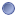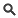### Module Detail Information

 Name: SPM fMRI Model SpecificationType:Module Short URL: `http://bit.ly/1AtBbv9` Description: Statistical analysis of fMRI data uses a mass-univariate approach based on General Linear Models (GLMs). Step1: specification of GLM matrix, fMRI data files and filtering. The design matrix defines the experimental design and the nature of hypothesis testing to be implemented. The design matrix has one row for each scan and one column for each effect or explanatory variable. (eg. regressor or stimulus function). You can build design matrices with separable session-specific partitions. Each partition may be the same (in which case it is only necessary to specify it once) or different. Responses can be either event- or epoch related, the only distinction is the duration of the underlying input or stimulus function. Mathematically they are both modeled by convolving a series of delta (stick) or box functions (u), indicating the onset of an event or epoch with a set of basis functions. These basis functions model the hemodynamic convolution, applied by the brain, to the inputs. This convolution can be first-order or a generalized convolution modeled to second order (if you specify the Volterra option). The same inputs are used by the Hemodynamic model or Dynamic Causal Models which model the convolution explicitly in terms of hidden state variables. Basis functions can be used to plot estimated responses to single events once the parameters (i.e. basis function coefficients) have been estimated. The importance of basis functions is that they provide a graceful transition between simple fixed response models (like the box-car) and finite impulse response (FIR) models, where there is one basis function for each scan following an event or epoch onset. The nice thing about basis functions, compared to FIR models, is that data sampling and stimulus presentation does not have to be synchronized thereby allowing a uniform and unbiased sampling of peri-stimulus time. Event-related designs may be stochastic or deterministic. Stochastic designs involve one of a number of trial-types occurring with a specified probability at successive intervals in time. These probabilities can be fixed (stationary designs) or time-dependent (modulated or non-stationary designs). The most efficient designs obtain when the probabilities of every trial type are equal. A critical issue in stochastic designs is whether to include null events If you wish to estimate the evoked response to a specific event type (as opposed to differential responses) then a null event must be included (even if it is not modeled explicitly). In SPM, analysis of data from multiple subjects typically proceeds in two stages using models at two 'levels'. The 'first level' models are used to implement a within-subject analysis. Typically there will be as many first level models as there are subjects. Analysis proceeds as described using the 'Specify first level' and 'Estimate' options. The results of these analyses can then be presented as 'case studies'. More often, however, one wishes to make inferences about the population from which the subjects were drawn. This is an example of a 'Random-Effects (RFX) analysis' (or, more properly, a mixed-effects analysis). In SPM, RFX analysis is implemented using the 'summary-statistic' approach where contrast images from each subject are used as summary measures of subject responses. These are then entered as data into a 'second level' model. Executable: `/spm8_batches/model_spec.sh` Input Parameters: - timing RT  - timing units  - input scans  - fmri_t  - fmri_t0  - cond_name  - cond_onset  - cond_dur  - tmod  - pmod_name  - pmod_param  - pmod_poly  - multi  - regress_name  - regress_val  - multi_reg  - hpf  - fact_name  - fact_levels  - hrf_derivs  - volt  - global  - mask  - cvi Output Parameters: - outdir File size: 33.64 KBView SourceDownloadOpen